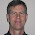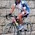## Monday, April 18, 2011

### Estimation of CdA from anthropometric data

by Andrew R. Coggan, Ph.D.

The popularity of wind tunnel testing to determine a cyclist's aerodynamic drag characteristics (i.e., their CdA, which is the product of their frontal area, A, and a dimensionless "shape factor", Cd) has grown considerably in recent years. A number of field tests for estimating CdA have also been developed and, in at least some cases, carefully validated/evaluated (e.g., http://www.trainingandracingwithapowermeter.com/2010/10/challenge-to-cycling-aerodynamicists.html). Nonetheless, there are times when a simple, "quick-and-dirty" estimate of someone's CdA is all that is needed/desired. For example, a cyclist or triathlete lacking a powermeter on their bike may still wish to estimate how much power they need to produce to achieve a particular performance, e.g., a certain average speed in a flat TT or triathlon bike leg. A convenient way of obtaining a ballpark estimate of CdA based upon easily-obtained measurements can also be used as a "smell test" to see whether other data (e.g., CdA values presented by others) make sense, and/or to place a given athlete's CdA in better context (i.e., are they more or less aero than typically found?). In such situations, it is possible to estimate A based on various anthropometric data, which can then be combined with an estimate of Cd to arrive at a final value for CdA. While this approach is rather crude, in my experience it works well enough to occasionally still be useful.

As indicated above, the first step is to estimate an individual's projected frontal area when in the aero position on their TT or triathlon bicycle. I am aware of at least five different formulae for making this calculation, as listed below. The first was originally related to me by Sam Callan, Director of Coaching Education for USA Cycling, whereas the other four are from the listed scientific papers. In the case of Heil's equations, STA = seat tube angle, TA = torso angle, and SW = shoulder width (readers of this blog are encouraged to consult the original paper to see precisely how these were defined/determined).

1. Australian Institute of Sport

Frontal area (m^2) = 0.18964 x height (m) + 0.00215 x mass (kg) - 0.07861
n = ?; R^2 = ?.??; P = ?.??; S.E.E. = ?.??? m^2

2. Bassett et al. (Med Sci Sports Exerc 1999; 31:1665-1676):

Frontal area (m^2) = 0.0293 x height (m) x mass (kg)^0.425 + 0.0604
n=8; R^2 = 0.76; P = 0.05; S.E.E. = 0.009 m^2

3. Heil DP. (Eur J Appl Physiol 2001; 85:358-366):

Frontal area (m^2) = [0.00433 x STA (deg)^0.172 x TA (deg)^0.096 x mass (kg)^0.762] + 0.066
n=21; R^2 = 0.54; P less than 0.001; S.E.E. = 0.017 m^2

Frontal area (m^2) = [0.00653 x STA (deg)^0.183 x TA (deg)^0.099 x mass (kg)^0.493 x height (m)^1.163] + 0.066
n=21; R^2 = 0.56; P less than 0.001; S.E.E. = 0.014 m^2

Frontal area (m^2) = [0.0148 x STA (deg)^0.184 x TA (deg)^0.099 x mass (kg)^0.408 x height (m)^0.925 x SW (m)^0.426] + 0.066
n=21; R^2 = 0.69; P less than 0.001; S.E.E. = 0.013 m^2

Once a value for frontal area is obtained, this must be multiplied by an appropriate value for Cd. Contrary to the assertions of many, cyclists are not "bluff bodies", i.e., the Cd of a cyclist upon a bicycle (even sitting upright on a mountain bike) is significantly less than that of, say, a flat plate, and perhaps more importantly, can vary as a function of yaw angle. Nonetheless, reasonable estimates of CdA (at 0 deg of yaw) can still usually be obtained by multiplying the above-derived frontal area(s) by 0.707, which is the average value for n=8 cyclists of varying stature and build tested by Dr. Chet Kyle in the Texas A&M wind tunnel (cf. Cycling Science 1991; Sept/Dec: 51-56 - Cd values ranged from 0.652 to 0.793). Alternatively, Cd can be estimated from body mass using an equation derived by Heil based on a meta-analysis of the literature:

Cd (unitless) = 4.45 x mass (kg)^-0.45

Given, however, the unknown precision of this equation and the fact that Kyle found no significant relationship between Cd and mass, there seems to be little reason to recommend it over simply using a fixed value of ~0.7.

So just how precisely can CdA be estimated using the approach described above? This question can be addressed two ways, i.e., via standard propogation-of-error analysis and also by example.

1) Propogation-of-error analysis: The standard errors of the estimate (S.E.E.) provided by each equation for estimating frontal area range range from 0.009 to 0.017 m^2. Frontal area, however, only accounts for ~60% of CdA, i.e., Cd can and does vary between individuals and thus accounts for the other ~40%. The S.E.E. values listed therefore do not tell the whole story, i.e., one must also take into consideration the variability in estimating Cd. Based on the data of Kyle and using standard propogation-of-error methods, the overall imprecision in estimating CdA would be 0.016-0.019 m^2, or plus/minus somewhere between 5 and 10% of a typical value.

2) Some examples: The table below lists anthropometric data along with estimates of A, Cd, and CdA obtained using the equations discussed above for two individuals, both of whom have been tested in the Texas A&M wind tunnel. Due in part to chance alone, the values obtained by using the third equation developed by Heil combined with either method of estimating Cd agree almost exactly with those determined in wind tunnel testing. While this outcome cannot be expected in all cases, the table below does serve to illustrate the range of values the various equations provide, and in fact it is often useful to "bracket" such estimates by calculating all possible outcomes (as shown), rather than relying upon just one single estimate.

Table 1. Estimates of A, Cd, and CdA using the various equations.1.BTW, if anyone would like a copy of the spreadsheet I used to generate Table 1, just email me!

2.May be a typo in the third equation?

[0.0148 x STA (deg)^0.184 ...

[0.00148 x STA (deg)^0.184 ...

3.Nice catch! Fortunately the spreadsheet I've been sending around is correct...

(EDIT: I've fixed the typo that bugno spotted, but will leave his comment up in case others have been misled by my mistake.)

4.Could you please send me a copy of the spreadsheet ?
Thank you so much.
m.bechis@repartosport.it

5.Yah, me too please. erblackiv@gmail.com

6.Massimo...it's easy to do

Note: Only a member of this blog may post a comment.